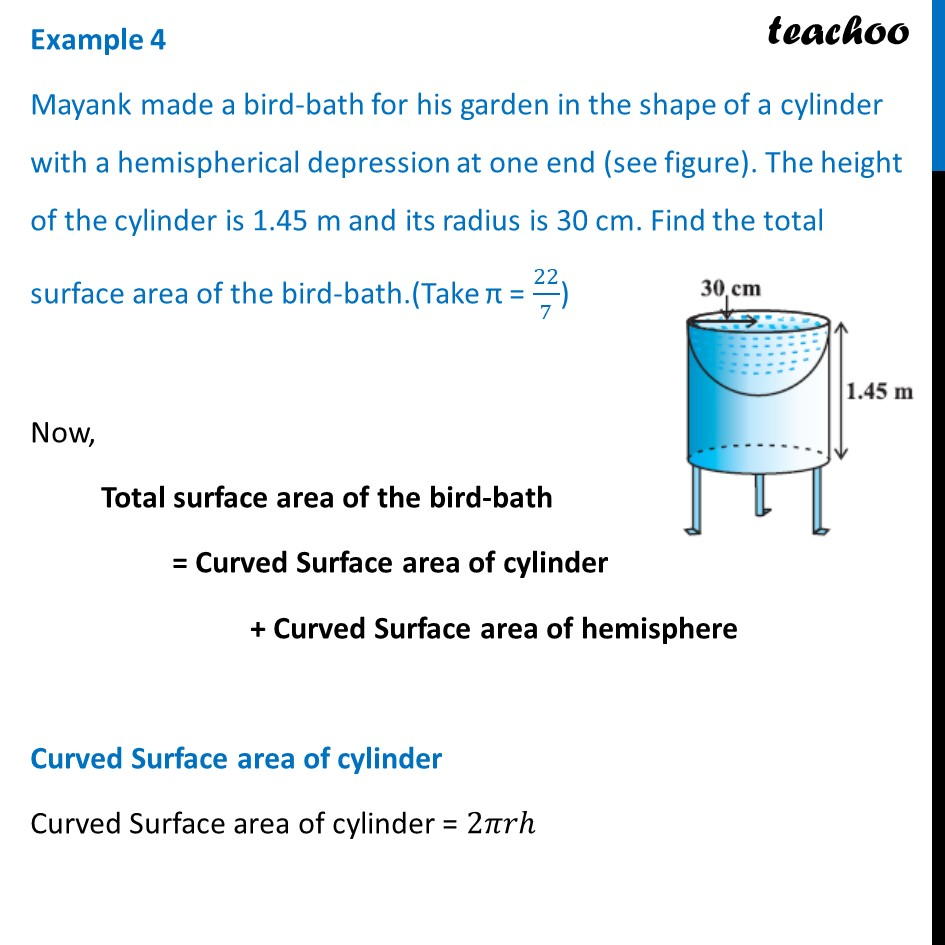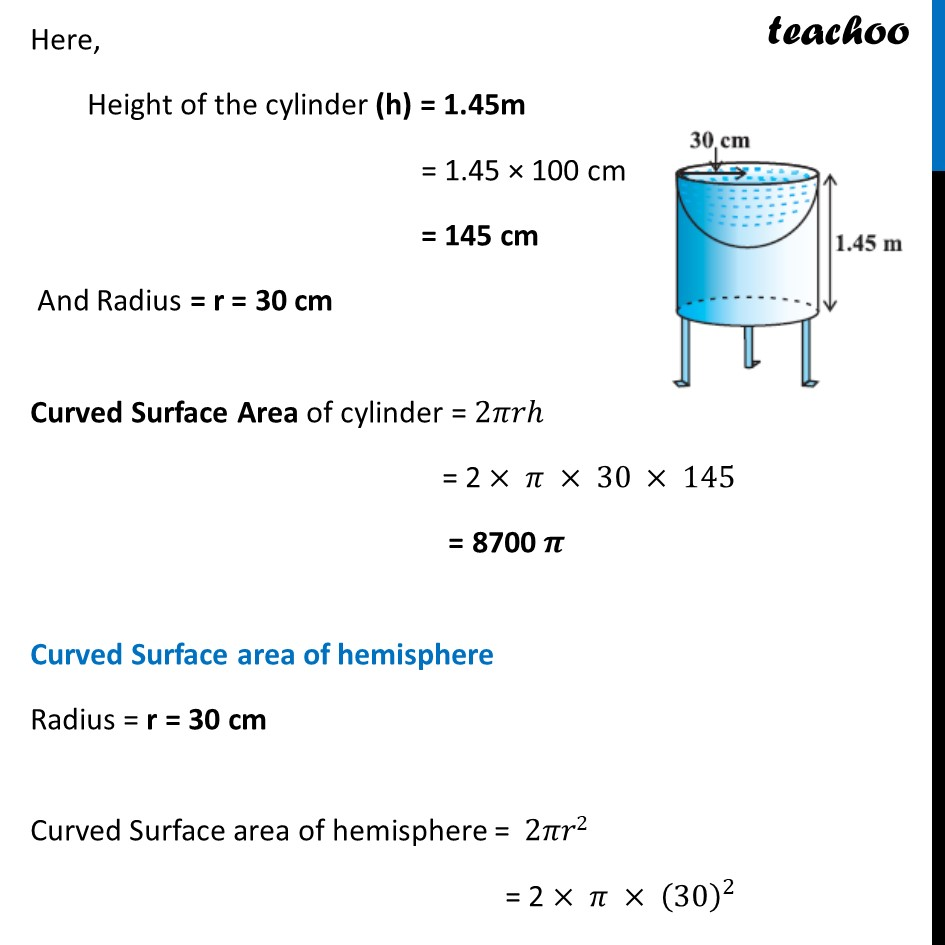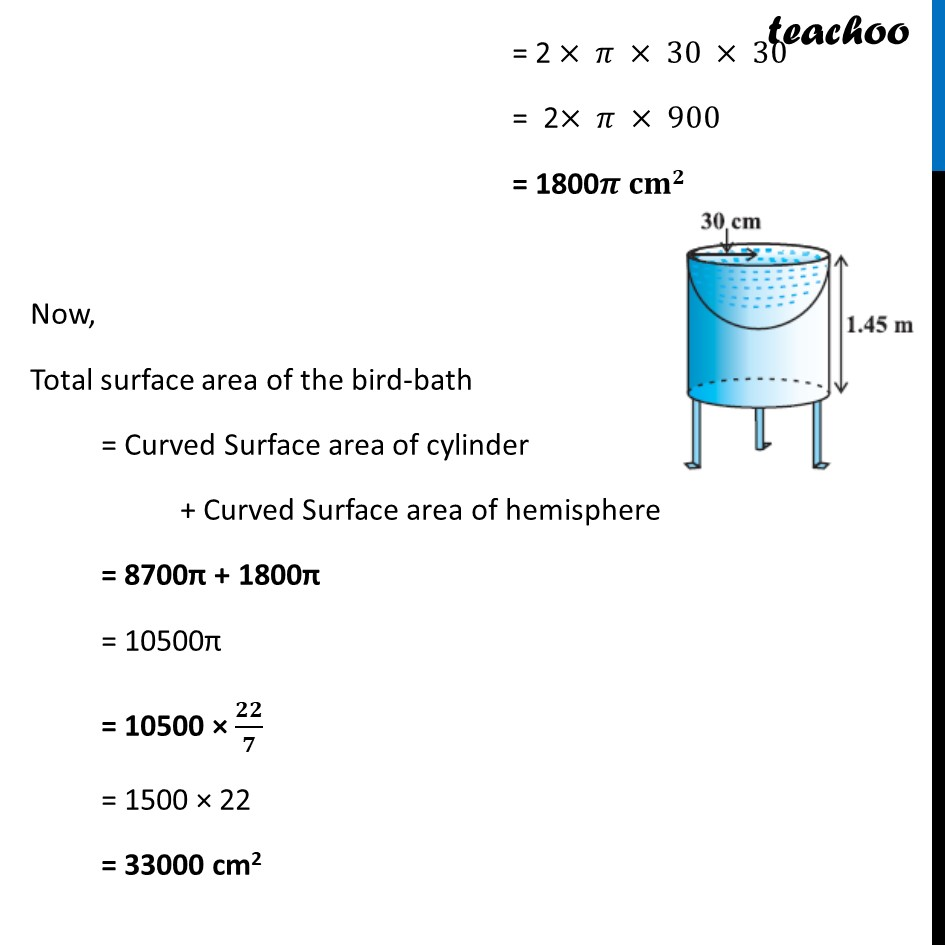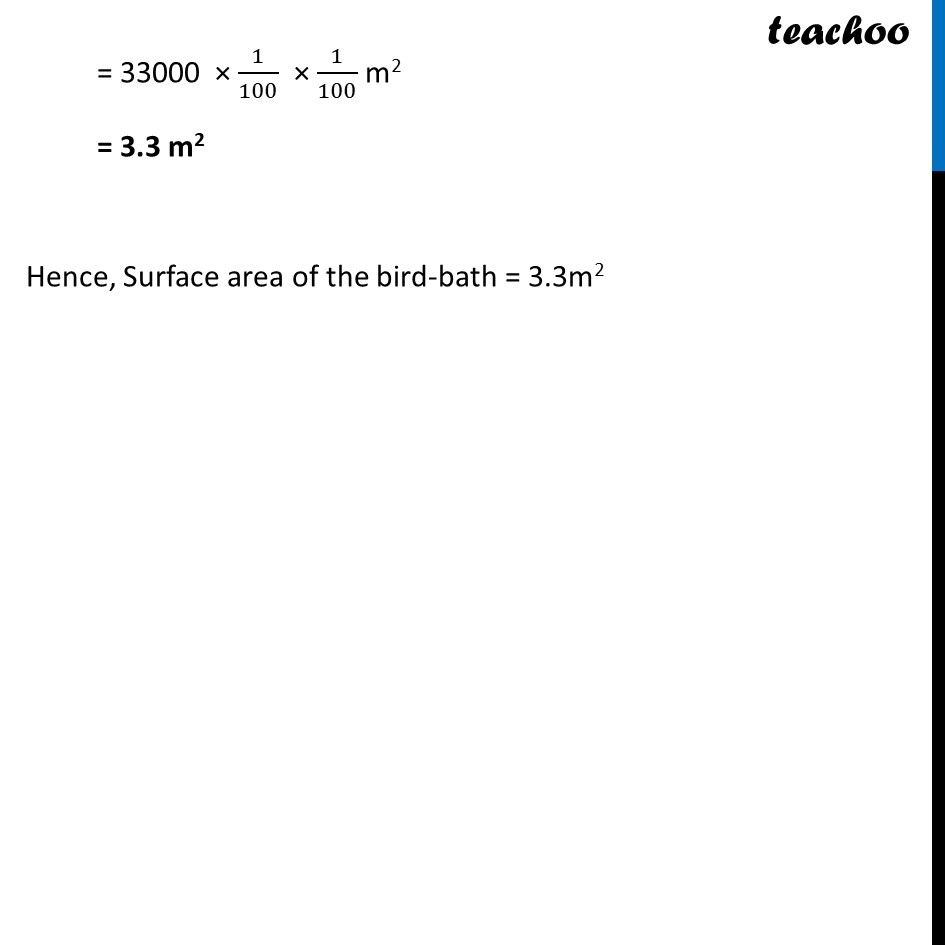Examples

Chapter 12 Class 10 Surface Areas and Volumes
Serial order wiseLearn in your speed, with individual attention - Teachoo Maths 1-on-1 Class

### Transcript

Example 4 Mayank made a bird-bath for his garden in the shape of a cylinder with a hemispherical depression at one end (see figure). The height of the cylinder is 1.45 m and its radius is 30 cm. Find the total surface area of the bird-bath.(Take π = 22/7) Now, Total surface area of the bird-bath = Curved Surface area of cylinder + Curved Surface area of hemisphere Curved Surface area of cylinder Curved Surface area of cylinder = 2𝜋𝑟ℎ Here, Height of the cylinder (h) = 1.45m = 1.45 × 100 cm = 145 cm And Radius = r = 30 cm Curved Surface Area of cylinder = 2𝜋𝑟ℎ = 2 × 𝜋 × 30 × 145 = 8700 𝝅 Curved Surface area of hemisphere Radius = r = 30 cm Curved Surface area of hemisphere = 2𝜋𝑟2 = 2 × 𝜋 × (30)^2 = 2 × 𝜋 × 30 × 30 = 2× 𝜋 × 900 = 1800𝝅 𝐜𝐦𝟐 Now, Total surface area of the bird-bath = Curved Surface area of cylinder + Curved Surface area of hemisphere = 8700π + 1800π = 10500π = 10500 × 𝟐𝟐/𝟕 = 1500 × 22 = 33000 cm2 = 33000 × 1/100 × 1/100 m2 = 3.3 m2 Hence, Surface area of the bird-bath = 3.3m2